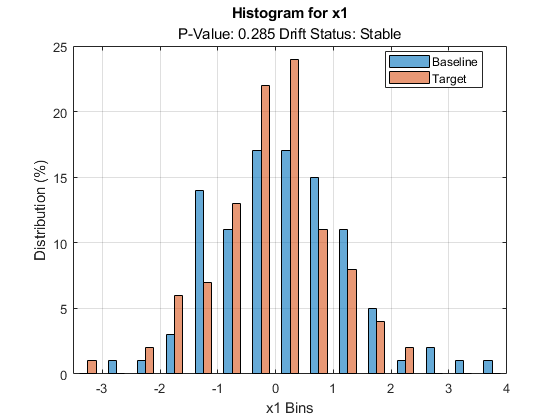# histcounts

Compute histogram bin counts for specified variables in baseline and target data for drift detection

## Syntax

``H = histcounts(DDiagnostics)``
``H = histcounts(DDiagnostics,Variables=variables)``

## Description

example

````H = histcounts(DDiagnostics)` returns the histogram bin counts in the table `H` for the variables specified for drift detection in the call to `detectdrift`.```

example

````H = histcounts(DDiagnostics,Variables=variables)` returns the bin counts for the variables specified by `variables`.```

## Examples

collapse all

Generate baseline and target data with two variables, where the distribution parameters of the second variable change for target data.

```rng('default') % For reproducibility baseline = [normrnd(0,1,100,1),wblrnd(1.1,1,100,1)]; target = [normrnd(0,1,100,1),wblrnd(1.2,2,100,1)];```

Perform permutation testing for any drift between the baseline and target data.

`DDiagnostics = detectdrift(baseline,target);`

Compute histogram bin counts for both variables.

`H = histcounts(DDiagnostics)`
```H=2×3 table Bins Counts_Baseline Counts_Target _____________________________________________________________________ ____________________________________________ __________________________________________ x1 {[-3.5000 -3 -2.5000 -2 -1.5000 -1 -0.5000 0 0.5000 1 1.5000 2 ... ]} {[0 1 1 3 14.0000 11 17 17 15 11 5 1 2 1 1]} {[1 0 2 6 7.0000 13 22 24 11 8 4 2 0 0 0]} x2 {[ 0 0.5000 1 1.5000 2 2.5000 3 3.5000 4 4.5000 5 5.5000 6]} {[ 33 23 14.0000 11 8 6 3 0 0 1 0 1]} {[ 13 32 29.0000 20 6 0 0 0 0 0 0 0]} ```

`H` is a table with three columns. `histcounts` divides the data into bins and computes the histogram bin counts for a variable in the baseline and target data over the common bins. The first and second rows have the bins and counts for variables `x1` and `x2`, respectively.

Access the histogram bin counts in baseline data for the first variable.

`H.Counts_Baseline{1}`
```ans = 1×15 0 1.0000 1.0000 3.0000 14.0000 11.0000 17.0000 17.0000 15.0000 11.0000 5.0000 1.0000 2.0000 1.0000 1.0000 ```

Plot the pdf estimate (percent data in each bin) of the baseline data for variable 1.

`histogram(BinEdges=H.Bins{1},BinCounts=H.Counts_Baseline{1},Normalization='probability')`You can also plot the histogram for baseline and target data for variable 1 using the `DriftDiagnostics` function.

`plotHistogram(DDiagnostics,Variable=1)``load humanactivity`

For details on the data set, enter `Description` at the command line.

Assign the first 1000 observations as baseline data and next 1000 as target data.

```baseline = feat(1:1000,:); target = feat(1001:2000,:);```

Test for drift on all variables.

`DDiagnostics = detectdrift(baseline,target);`

Compute the histogram bin counts for only the first five variables.

`H = histcounts(DDiagnostics,Variables=(1:5))`
```H=5×3 table Bins Counts_Baseline Counts_Target ___________________________________________________________________________ ______________________________________________________________________ ___________________________________________________________________________ x1 {[-0.2000 -0.1000 0 0.1000 0.2000 0.3000 0.4000 0.5000 0.6000 0.7000 ... ]} {[ 0 0 0 0 0 0 0 0 0 85.9000 14.1000]} {[ 12.4000 76.6000 2.1000 0 0 0 0.1000 0.1000 0.1000 0.1000 8.5000]} x2 {[ -0.3000 -0.2000 -0.1000 0 0.1000 0.2000 0.3000 0.4000 0.5000 ... ]} {[ 0 0 0 0 0 9.9000 24 0.3000 65.8000 0 0 0 0 0]} {[ 0.1000 0 0.1000 0.1000 0.1000 8.2000 0.3000 0 0 0 0 0 53.8000 37.3000]} x3 {[ -0.6000 -0.5500 -0.5000 -0.4500 -0.4000 -0.3500 -0.3000 -0.2500 ... ]} {[0 19.9000 13.6000 0.3000 0.3000 0.2000 65.7000 0 0 0 0 0 0 0 0 0 0]} {[0.1000 0.4000 8.4000 0 0 0 0 0 12.9000 4.1000 0.3000 0.2000 0.4000 ... ]} x4 {[ 0 0.0100 0.0200 0.0300 0.0400 0.0500 0.0600 0.0700 0.0800 0.0900 ... ]} {[ 0 0 0 0 0 0 0 0 0 0 65.6000 33.9000 0.4000 0.1000 0 0 0 0 0 0]} {[34.5000 55.7000 0.9000 0 0 0 0 0 0 0 0 7.4000 0.5000 0.2000 0.3000 ... ]} x5 {[ 0.0300 0.0400 0.0500 0.0600 0.0700 0.0800 0.0900 0.1000 0.1100 ... ]} {[ 0.3000 33.1000 0 0 0.3000 66 0.3000 0 0 0 0 0 0 0]} {[0 7.5000 0.5000 0.1000 0 0 0 0.1000 0.1000 0 0.2000 91.1000 0.2000 ... ]} ```

Access the histogram bin counts for the second variable in target data.

`H.Counts_Target{2}`
```ans = 1×14 0.1000 0 0.1000 0.1000 0.1000 8.2000 0.3000 0 0 0 0 0 53.8000 37.3000 ```

## Input Arguments

collapse all

Diagnostics of the permutation testing for drift detection, specified as a `DriftDiagnostics` object returned by `detectdrift`.

List of variables for which to compute the histogram bin counts, specified as a string array, a cell array of character vectors, or a list of integer indices.

Example: `Variables=["x1","x3"]`

Example: `Variables=(1,3)`

Data Types: `single` | `double` | `char` | `string`

## Output Arguments

collapse all

Histogram bin counts, returned as a table with the following columns.

Column NameDescription
Bins

Common domain over which to evaluate the histogram bin counts for a variable.

• For categorical variables, `Bins` contains the categories.

• For continuous variables, `Bins` contains the bin edges.

Count_BaselineHistogram bin counts for the corresponding variables in baseline data.
Counts_TargetHistogram bin counts for the corresponding variables in target data.

For each variable in `H`, the columns hold the bins and the counts in cell arrays. To access the counts, you can index into the table; for example, to obtain the histogram bin counts for the second variable in baseline data, use `H.Counts_Baseline{2,1}`.

## Algorithms

• For categorical data, `detectdrift` adds 0.5 correction factor to histogram bin counts for each bin to handle empty bins (categories). This is equivalent to the assumption that the parameter p, probability that value of the variable would be in that category, has the prior distribution Beta(0.5,0.5), i.e. Jefferys prior assumption for the distribution parameter.

• `histcounts` treats a variable as ordinal for visualization purposes under any of the following cases:

• If the variable is ordinal in either baseline or target data and the categories from baseline and target data are the same.

• If the variable is ordinal in either in baseline or target data and the categories of the other data set is a subset of the ordinal data.

• If the variable is ordinal in both baseline and target data and categories from either one is a subset of the other.

• If a variable is ordinal, `histcounts` preserves the order of the bin names.

## Version History

Introduced in R2022a